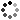# Mathematical Artwork in Python Ebook + Extras

On Sale
\$40.00
\$40.00
Learn how to write Python code to create mathematically-based artwork and fractals. This 206-page PDF and EPUB e-book provides simple Python code, relying only on common libraries such as Numpy, Pillow, and Matplotlib, so the reader can fully understand the math behind the images. The book intends to bridge the gap between math, code, and design. The book assumes a basic knowledge of Python and some high-school level math.

This product includes the PDF and EPUB book plus the Extras Pack:
• Jupyter Notebooks with all code in the book
• Jupyter notebook with interactive-widgets for exploring Mandelbrot and Julia Sets. Click-and-drag to pan and zoom, all from a Jupyter notebook.
• Interactive-widgets notebook for exploring Lissajous curves, harmonograph equation drawings, and spirograph equation drawings.
• Interactive-widgets notebook for creating and exploring strange attractor plots.
• Code for generating FFT-based sketches (in the style of Wolfram), and their parametric equations, by point-and-click.
• Jupyter notebook for interactively creating color palettes
• A list of 400 points in the complex plane, listed to 17 digits of precision, that iterate the Mandelbrot equation at least 1 million and up to 5 million times before escaping. Save your CPU when making high-resolution Buddhabrot fractals.
License: Any original work you create using the code and ideas in the book are your own and may be sold or distributed as you like. The book, code, and all other contents of this product may not be resold or distributed.
You will get the following files:
• PDF (15MB)
• EPUB (18MB)
• ZIP (18MB)
• ZIP (448KB)